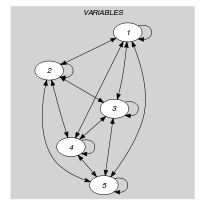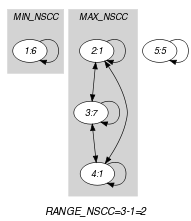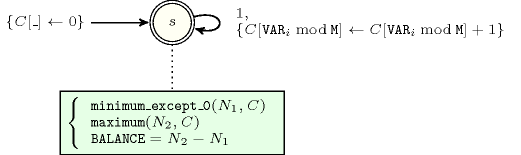## 5.46. balance_modulo

Origin
Constraint

$\mathrm{𝚋𝚊𝚕𝚊𝚗𝚌𝚎}_\mathrm{𝚖𝚘𝚍𝚞𝚕𝚘}\left(\mathrm{𝙱𝙰𝙻𝙰𝙽𝙲𝙴},\mathrm{𝚅𝙰𝚁𝙸𝙰𝙱𝙻𝙴𝚂},𝙼\right)$

Arguments
 $\mathrm{𝙱𝙰𝙻𝙰𝙽𝙲𝙴}$ $\mathrm{𝚍𝚟𝚊𝚛}$ $\mathrm{𝚅𝙰𝚁𝙸𝙰𝙱𝙻𝙴𝚂}$ $\mathrm{𝚌𝚘𝚕𝚕𝚎𝚌𝚝𝚒𝚘𝚗}\left(\mathrm{𝚟𝚊𝚛}-\mathrm{𝚍𝚟𝚊𝚛}\right)$ $𝙼$ $\mathrm{𝚒𝚗𝚝}$
Restrictions
 $\mathrm{𝙱𝙰𝙻𝙰𝙽𝙲𝙴}\ge 0$ $\mathrm{𝙱𝙰𝙻𝙰𝙽𝙲𝙴}\le \mathrm{𝚖𝚊𝚡}\left(0,|\mathrm{𝚅𝙰𝚁𝙸𝙰𝙱𝙻𝙴𝚂}|-2\right)$ $\mathrm{𝚛𝚎𝚚𝚞𝚒𝚛𝚎𝚍}$$\left(\mathrm{𝚅𝙰𝚁𝙸𝙰𝙱𝙻𝙴𝚂},\mathrm{𝚟𝚊𝚛}\right)$ $𝙼>0$
Purpose

Consider the largest set ${𝒮}_{1}$ (respectively the smallest set ${𝒮}_{2}$) of variables of the collection $\mathrm{𝚅𝙰𝚁𝙸𝙰𝙱𝙻𝙴𝚂}$ that have the same remainder when divided by $𝙼$. $\mathrm{𝙱𝙰𝙻𝙰𝙽𝙲𝙴}$ is equal to the difference between the cardinality of ${𝒮}_{2}$ and the cardinality of ${𝒮}_{1}$.

Example
$\left(2,〈6,1,7,1,5〉,3\right)$

In this example values 6, 1, 7, 1, 5 are respectively associated with the equivalence classes $6\mathrm{mod}3=0$, $1\mathrm{mod}3=1$, $7\mathrm{mod}3=1$, $1\mathrm{mod}3=1$, $5\mathrm{mod}3=2$. Therefore the equivalence classes 0, 1 and 2 are respectively used 1, 3 and 1 times. The $\mathrm{𝚋𝚊𝚕𝚊𝚗𝚌𝚎}_\mathrm{𝚖𝚘𝚍𝚞𝚕𝚘}$ constraint holds since its first argument $\mathrm{𝙱𝙰𝙻𝙰𝙽𝙲𝙴}$ is assigned to the difference between the maximum and minimum number of the previous occurrences (i.e., $3-1$).

Typical
 $|\mathrm{𝚅𝙰𝚁𝙸𝙰𝙱𝙻𝙴𝚂}|>2$ $𝙼>1$ $𝙼<$$\mathrm{𝚖𝚊𝚡𝚟𝚊𝚕}$$\left(\mathrm{𝚅𝙰𝚁𝙸𝙰𝙱𝙻𝙴𝚂}.\mathrm{𝚟𝚊𝚛}\right)$
Symmetries
• Items of $\mathrm{𝚅𝙰𝚁𝙸𝙰𝙱𝙻𝙴𝚂}$ are permutable.

• An occurrence of a value $u$ of $\mathrm{𝚅𝙰𝚁𝙸𝙰𝙱𝙻𝙴𝚂}.\mathrm{𝚟𝚊𝚛}$ can be replaced by any other value $v$ such that $v$ is congruent to $u$ modulo $𝙼$.

Arg. properties

Functional dependency: $\mathrm{𝙱𝙰𝙻𝙰𝙽𝙲𝙴}$ determined by $\mathrm{𝚅𝙰𝚁𝙸𝙰𝙱𝙻𝙴𝚂}$ and $𝙼$.

Usage

An application of the $\mathrm{𝚋𝚊𝚕𝚊𝚗𝚌𝚎}_\mathrm{𝚖𝚘𝚍𝚞𝚕𝚘}$ constraint is to enforce a balanced assignment of values, no matter how many distinct equivalence classes will be used. In this case one will push down the maximum value of the first argument of the $\mathrm{𝚋𝚊𝚕𝚊𝚗𝚌𝚎}_\mathrm{𝚖𝚘𝚍𝚞𝚕𝚘}$ constraint.

specialisation: $\mathrm{𝚋𝚊𝚕𝚊𝚗𝚌𝚎}$ ($\mathrm{𝚟𝚊𝚛𝚒𝚊𝚋𝚕𝚎}\mathrm{mod}\mathrm{𝚌𝚘𝚗𝚜𝚝𝚊𝚗𝚝}$ replaced by $\mathrm{𝚟𝚊𝚛𝚒𝚊𝚋𝚕𝚎}$).

Keywords
Arc input(s)

$\mathrm{𝚅𝙰𝚁𝙸𝙰𝙱𝙻𝙴𝚂}$

Arc generator
$\mathrm{𝐶𝐿𝐼𝑄𝑈𝐸}$$↦\mathrm{𝚌𝚘𝚕𝚕𝚎𝚌𝚝𝚒𝚘𝚗}\left(\mathrm{𝚟𝚊𝚛𝚒𝚊𝚋𝚕𝚎𝚜}\mathtt{1},\mathrm{𝚟𝚊𝚛𝚒𝚊𝚋𝚕𝚎𝚜}\mathtt{2}\right)$

Arc arity
Arc constraint(s)
$\mathrm{𝚟𝚊𝚛𝚒𝚊𝚋𝚕𝚎𝚜}\mathtt{1}.\mathrm{𝚟𝚊𝚛}\mathrm{mod}𝙼=\mathrm{𝚟𝚊𝚛𝚒𝚊𝚋𝚕𝚎𝚜}\mathtt{2}.\mathrm{𝚟𝚊𝚛}\mathrm{mod}𝙼$
Graph property(ies)
$\mathrm{𝐑𝐀𝐍𝐆𝐄}_\mathrm{𝐍𝐒𝐂𝐂}$$=\mathrm{𝙱𝙰𝙻𝙰𝙽𝙲𝙴}$

Graph class
$\mathrm{𝙴𝚀𝚄𝙸𝚅𝙰𝙻𝙴𝙽𝙲𝙴}$

Graph model

The graph property $\mathrm{𝐑𝐀𝐍𝐆𝐄}_\mathrm{𝐍𝐒𝐂𝐂}$ constraints the difference between the sizes of the largest and smallest strongly connected components.

Parts (A) and (B) of Figure 5.46.1 respectively show the initial and final graph associated with the Example slot. Since we use the $\mathrm{𝐑𝐀𝐍𝐆𝐄}_\mathrm{𝐍𝐒𝐂𝐂}$ graph property, we show the largest and smallest strongly connected components of the final graph.

##### Figure 5.46.1. Initial and final graph of the $\mathrm{𝚋𝚊𝚕𝚊𝚗𝚌𝚎}_\mathrm{𝚖𝚘𝚍𝚞𝚕𝚘}$ constraint(a) (b)
Automaton

Figure 5.46.2 depicts the automaton associated with the $\mathrm{𝚋𝚊𝚕𝚊𝚗𝚌𝚎}_\mathrm{𝚖𝚘𝚍𝚞𝚕𝚘}$ constraint. To each item of the collection $\mathrm{𝚅𝙰𝚁𝙸𝙰𝙱𝙻𝙴𝚂}$ corresponds a signature variable ${S}_{i}$ that is equal to 1.

##### Figure 5.46.2. Automaton of the $\mathrm{𝚋𝚊𝚕𝚊𝚗𝚌𝚎}_\mathrm{𝚖𝚘𝚍𝚞𝚕𝚘}$ constraint